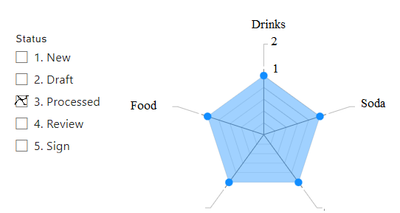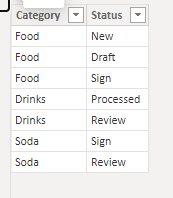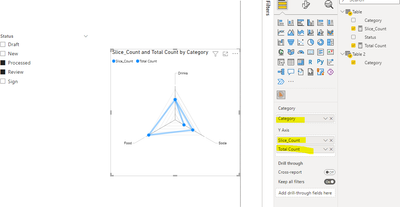cancel
Showing results for
Did you mean:Helper I

## Filter a Radar Chart using a slicer

I have the following table:I need to show this data in a Radar Chart, and filter it using a Slicer as such:When a Slicer filter is clicked such as "3. Processed", the Radar Chart should show how many of each category have the status "3. Processed".

It should display the actual amount (in this case, 1), but also show the total amount of Status for each Category (in this case, 2, since "Drinks" has a total of 2 rows). We should not count distinct rows only, so if there was one more status called "Draft" for "Drinks", then the total would be 3.

How to achieve this?

1 ACCEPTED SOLUTIONCommunity Support

Hi , @msuser48

According to your description , you want to Filter a Radar Chart using a slicer. RIght?

Here are the steps you can follow:

(1)This is my test data which is the same as yours:(2)We can click "New Table" to create a table:

``Table 2 = ALL('Table'[Category])``

(3)We can create two measures:

``````Total Count =
var _current_ca= SELECTEDVALUE('Table 2'[Category])
var _t= FILTER( ALL('Table'),'Table'[Category]=_current_ca)
return COUNTROWS(_t)``````
``````Slice_Count = var _slice = VALUES('Table'[Status])
var _current_ca= SELECTEDVALUE('Table 2'[Category])
var _t = FILTER( ALL('Table') ,'Table'[Status] in _slice && 'Table'[Category] = _current_ca)
return
COUNTROWS(_t)``````

(4)Then we can put the two measure and the 'Table2'[Category] in the Radar visual and we can meet your need, the result is as follows:If this method does not meet your needs, you can provide us with your special sample data and the desired output sample data in the form of tables, so that we can better help you solve the problem.

Best Regards,

Aniya Zhang

If this post helps, then please consider Accept it as the solution to help the other members find it more quicklyCommunity Support

Hi , @msuser48

According to your description , you want to Filter a Radar Chart using a slicer. RIght?

Here are the steps you can follow:

(1)This is my test data which is the same as yours:(2)We can click "New Table" to create a table:

``Table 2 = ALL('Table'[Category])``

(3)We can create two measures:

``````Total Count =
var _current_ca= SELECTEDVALUE('Table 2'[Category])
var _t= FILTER( ALL('Table'),'Table'[Category]=_current_ca)
return COUNTROWS(_t)``````
``````Slice_Count = var _slice = VALUES('Table'[Status])
var _current_ca= SELECTEDVALUE('Table 2'[Category])
var _t = FILTER( ALL('Table') ,'Table'[Status] in _slice && 'Table'[Category] = _current_ca)
return
COUNTROWS(_t)``````

(4)Then we can put the two measure and the 'Table2'[Category] in the Radar visual and we can meet your need, the result is as follows:If this method does not meet your needs, you can provide us with your special sample data and the desired output sample data in the form of tables, so that we can better help you solve the problem.

Best Regards,

Aniya Zhang

If this post helps, then please consider Accept it as the solution to help the other members find it more quickly